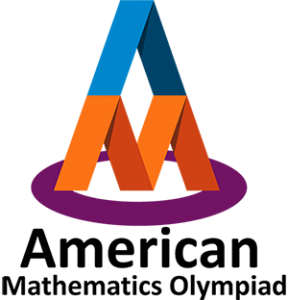You can use Edugain to prepare for AMO (American Mathematics Olympiad) exam using unlimited printable worksheets, online practice and online tests. For official information about AMO, visit amo.sg

American Mathematics Olympiad (AMO) is an affiliate of Math Olympiads for Elementary and Middle Schools, USA (MOEMS) and there are similar affiliates in Columbia, Iceland, Australia (APSMO), Philippines, China and India.

*Edugain has no affiliation with AMO or amo.sg. Edugain is a learning platform to help students prepare well for the exam.
Mixed Operations, Logical Reasoning, Time, Shapes and Patterns, Large Numbers, Geometry, Multiplication, Roman Numerals, Division, Fractions, Measurement - Length, Mass & Capacity, Natural and Whole Numbers, Mensuration - Perimeter, Area, Volume, etc., Money Transactions, Symmetry, Graphs, Number System, Basic, Quadrilaterals, Numbers, Century Year, Tangram, Pictograph, Tally Marks, Data Handling

Large Numbers, Roman Numerals, Multiplication, Division, Mixed Operations, Unitary Method, Geometry, Natural and Whole Numbers, Fractions, Measurement - Length, Mass & Capacity, Decimals, Time, Mensuration - Perimeter, Area, Volume, etc., Logical Reasoning, Money Transactions, Symmetry, Graphs, Numbers, Tangram, Tiling, estimation, Data Handling

Large Numbers, Roman Numerals, Multiplication, Division, Mixed Operations, Unitary Method, Natural and Whole Numbers, Fractions, Decimals, Geometry, Measurement - Length, Mass & Capacity, Ratio & Proportions, Percentages, Time, Money Transactions, Mensuration - Perimeter, Area, Volume, etc., Logical Reasoning, Number System, Computation Operations, Data Handling, Graphs, Numbers, Pictograph, Simplification

Large Numbers, Natural and Whole Numbers, Integers, Fractions, Decimals, Ratio & Proportions, Percentages, Geometry, Mensuration - Perimeter, Area, Volume, etc., Algebra, Data Handling, Logical Reasoning, Unitary Method, Symmetry, Number System, Linear Equations In One Variable, Simplification, Triangles, Quadratic Equations, Numbers, Cubes and Cube Root

Algebra, Integers, Symmetry, Data Handling, Logical Reasoning, Fractions, Mensuration - Perimeter, Area, Volume, etc., Geometry, Exponents and Powers, Decimals, Rational Numbers, Percentages, Ratio & Proportions, Congruence of Triangles, Trigonometry, Numbers

Algebra, Rational Numbers, Square and Square Roots, Graphs, Cubes and Cube Root, Exponents and Powers, Mensuration - Perimeter, Area, Volume, etc., Data Handling, Unitary Method, Percentages, Logical Reasoning, Natural and Whole Numbers, Linear Equations In One Variable, Geometry, Ratio & Proportions, Integers, Symmetry, Comparing Quantities, Polygons, Fractions, Multiplication, Number System, Numbers